Next Continued Fraction Arithmetic 37

# Continued fraction arithmetic

• Instead of z = z(x), we will have z = z(x, y)

• Instead of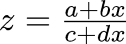we will have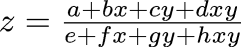• We will abbreviate this to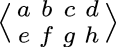• z can do three things:

• Input a term p from x: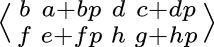• Input a term q from y: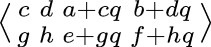• Output a term r: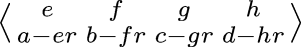• Summary:

•is quite similar to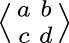, but with two variables instead of one

 Next Back